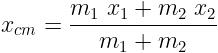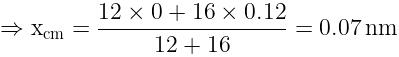Courses

# Test: Centre of Mass- 1

## 20 Questions MCQ Test Physics For JEE | Test: Centre of Mass- 1

Description
This mock test of Test: Centre of Mass- 1 for JEE helps you for every JEE entrance exam. This contains 20 Multiple Choice Questions for JEE Test: Centre of Mass- 1 (mcq) to study with solutions a complete question bank. The solved questions answers in this Test: Centre of Mass- 1 quiz give you a good mix of easy questions and tough questions. JEE students definitely take this Test: Centre of Mass- 1 exercise for a better result in the exam. You can find other Test: Centre of Mass- 1 extra questions, long questions & short questions for JEE on EduRev as well by searching above.
QUESTION: 1

### There are two objects of masses 1 kg and 2 kg located at (1, 2) and (-1, 3) respectively. The coordinates of the centre of mass are

Solution:

Body A has mass of 1kg and location (1,2)
Body B has mass of 2kg and location (-1,3)

mcxc = m1x1 + m2x2
(1+2) xc = (1 * 1) + (2 * -1)
xc  = -1/3

Similarly,
mcyc = m1y1 + m2y2
(1 + 2) yc = (1 * 2) + (2 * 3)
yc= 8/3

Hence, the coordinates of the center of mass are (-1/3, 8/3).

QUESTION: 2

### The motion of a potter’s wheel is an example of

Solution:

Potter’s wheel is an example of rotary motion. Rotary motion is that kind of motion in which body of the mass moves along a circular path about an axis which remains fixed.

QUESTION: 3

### The general motion of a rigid body consists of

Solution:

The general motion of a rigid body consists of both, translational and rotational motion. This is much obvious as it is seen all around us.

QUESTION: 4

A rigid body is one

Solution:

A rigid body is one in which the distance between all pairs of particles remains fixed.

QUESTION: 5

If a shell at rest explodes then the centre of mass of the fragments

Solution:

As a shell explosion is not governed by any external force, we get that no external force acts upon the shell, and hence it remains at rest.

QUESTION: 6

A child sits stationary at one end of long trolley moving uniformly with speed v on a smooth horizontal floor. If the child gets up and runs about on the trolley in the forward direction with speed u. The centre of mass of the system (child + trolley) will move with speed

Solution:

The child is running arbitrarily on a trolley moving with velocity v.
However, the running of the child will produce no effect on the velocity of the centre of mass of the trolley.
This is because the force due to the boy’s motion is purely internal.

Internal forces produce no effect on the motion of the bodies on which they act.

Since no external force is involved in the boy–trolley system, the boy’s motion will produce no change in the velocity of the centre of mass of the trolley.

QUESTION: 7

There are some passengers inside a stationary railway compartment. The centre of masses of the compartment itself(without the passengers) is C1, while the centre of mass of the compartment plus passengers’ system is C2. if the passengers moves about inside the compartment

Solution:

Given the track is frictionless, therefore as the people inside will move inside the compartment the compartment will itself move opposite to it.
Hence C1 will move but as their is no external force on the combined system and its initial velocity is also zero. Therefore C2 won't change.

QUESTION: 8

If a man of mass M jumps to the ground from height h and his centre of mass moves a distance x in the time taken by him to hit the ground, the average force acting on him is (assuming his retardation to be constant during his impact with the ground)

Solution:

As the center of mass moves through a distance x, the average force F acting on the man is calculated from :
Work done = Change in potential energy
⇒ Fx = Mgh
⇒ F =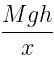QUESTION: 9

The centre of mass of a body is located

Solution:

The centre of mass of a body can lie within or outside the body.
Example
(i)
Centre of mass of a uniform rod lies at its geometrical centre which lies within the rod
(ii) Centre of mass of a uniform ring lies at its geometrical centre which lies outside the ring.

QUESTION: 10

When a shell was following a parabolic path in the air, it explodes somewhere in its flight. The center of mass of fragments will continue to move in

Solution:

The internal forces have no effect on the trajectory of the center of mass, and the forces due to explosion are the internal forces. So the center of mass will follow the same parabolic path even after the explosion.

QUESTION: 11

A mass m is moving with a constant velocity along a line parallel to the x-axis, away from the origin. Its angular momentum with respect to the origin

Solution:

Angular momentum (L) is defined as the distance of the object from a rotation axis multiplied by the linear momentum
L = mv×h

As the particle moves, m; v; and h, all remain unchanged at any point of time
⇒ L = constant

QUESTION: 12

Every point in a rotating rigid body has the same __________at any instant of time.

Solution:

Every point in any rigid rotating object is rotating at the same angular velocity. However, I have seen a few cases where people have used the term "angular velocity" when they really meant tangential velocity, so you do have to be careful.

QUESTION: 13

An isolated particle of mass m is moving in a horizontal plane (x,y) along the x axis at a certain height above the ground. It suddenly explodes into two fragments of masses m/4 and 3m/4. An instant later, the smaller fragments is at y = +15 cm. The larger fragment at this instant is at

Solution:

As the particle is exploded only due to its internal energy.
net external force during this process is 0 i.e. center mass will not change.

Let the particle while the explosion was above the origin of the coordinate system i.e. just before explosion xcm =0 and ycm =0

After the explosion, the Centre of mass will be at xcm =0 and ycm =0

Since smaller fragment has fallen on the y-axis.
Let positon of larger fragment be y.

m * ycm = (m/4 * 15) + (3m/4 * y)
⇒ (m/4 * 15) + (3m/4 * y) = 0
⇒ y = - 5 cm

QUESTION: 14

The centre of mass of a system of particles does not depend on

Solution:

The resultant of all forces, on any system of particles, is zero. Therefore, their centre of mass does not depend upon the forces acting on the particles.

QUESTION: 15

If the net force acting on the system of particles is zero, then which of the following may vary

Solution:
QUESTION: 16

If three balls of same radius are placed touching each other on a horizontal surface such that they will form an equilateral triangle, when their centers are joined. What will be the position of the centre of mass of the system?

Solution:

The COM will be at the intersection point of the medians as that is the most symmetric point of this symmetric system.

QUESTION: 17

When external forces acting on a body are zero, then its centre of mass

Solution:

When force acting upon the body results zero, the resulting acceleration due to net force applied is also zero, and hence by the law of inertia the motion of the body either at rest or constant velocity wont change.

QUESTION: 18

Two blocks of the masses 10 kg and 4 kg are connected by a spring of negligible mass and placed on a frictionless horizontal surface. An impulse gives a velocity of 14 m/s to the heavier block. The velocity of centre of mass is

Solution:

Velocity of heavier block (v2) = 14m/s
Velocity of lighter block (v1) = 0m/s
Velocity of centre of mass,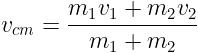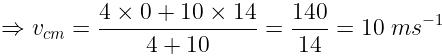QUESTION: 19

The sum of moments of masses of all the particles in a system about the centre of mass is always:

Solution:

Using,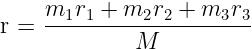Let origin be the centre of mass of the body which is represented by C i.e. r = 0
⟹ m1r+ m2​r+ m3r= 0

Now net moment about C,
τC = (m1g) * r1 + (m2g) * r+ (m3g) * r3
τC = g [m1r+ m2​r+ m3r3] = 0

Hence, the sum of moment of masses of all the particles in the system about COM is always Zero

QUESTION: 20

In a CO molecule, the distance between carbon and oxygen atom is 0.12 nm. What is the distance of centre of mass from the carbon atom?

Solution:

The distance of centre of mass from carbon atom,